Courses
Courses for Kids
Free study material
Offline Centres
MoreLast updated date: 01st Dec 2023
Total views: 279k
Views today: 6.79k

# Which rectangle A, B, C or D fits into the big square without turning?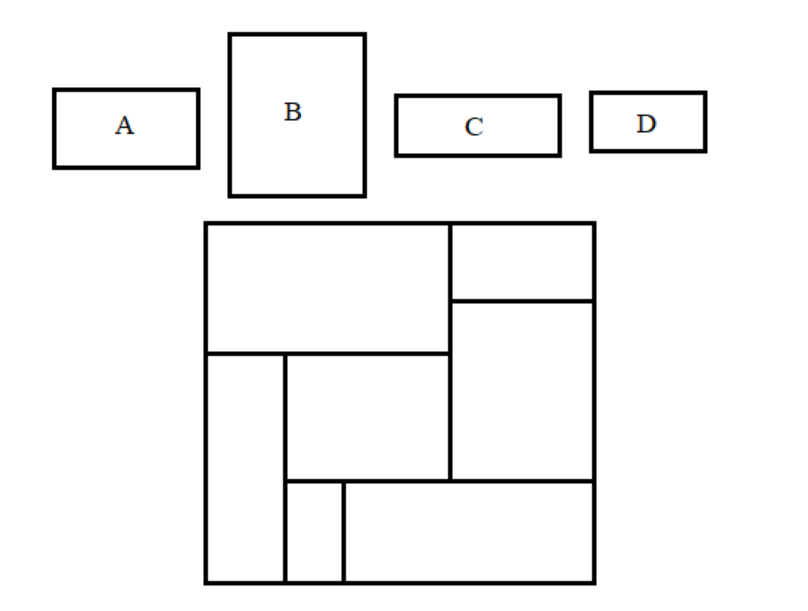Verified
279k+ views
Hint: We all have played Puzzle games, and that is the base for this question, as you can see that parts are to be fixed in the big main square board. In this case we also have to give a look at the length and breadth of the rectangles, whether it fits the length and breadth of the rectangles in the main square board. Also, they have given a restriction that parts cannot be rotated, we have to fix it as it is.

In this problem our aim is to find which of the given rectangles fits into the bid square without rotating it. We first let us take option (a) A.
That is,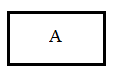When we see the length and breadth of this rectangle A, its matches a place in the big square picture.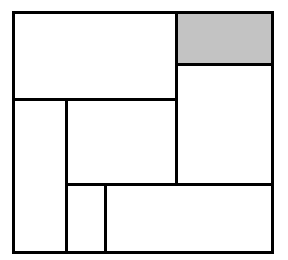Thus, without rotating the rectangle A, we can fit it into the shaded part of the big square, as its length and breadth looks equal.
Let us take the next option (b) B. That is,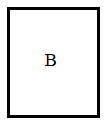Even this rectangle B can also look as equal as the shaded portion of the big square. That is,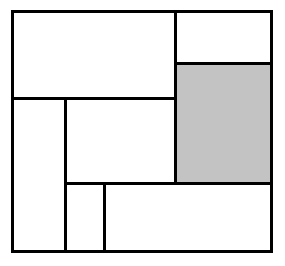But the breadth of rectangle B is smaller than the length of the rectangle part in the big square. Thus, we cannot fit rectangle B into it.
Let us see the next option (c) C. That is,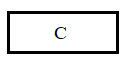It looks the same as the shaded portion of the big square.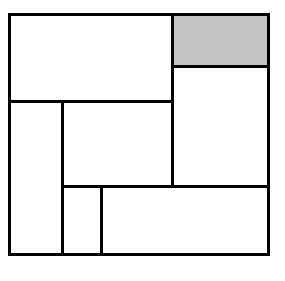But the length of the rectangle C doesn’t fit to the shaded rectangle part of the big square.
Let’s see the last option (d) D. that is,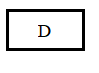It can be fixed in the shaded portion of the big square if it is rotated.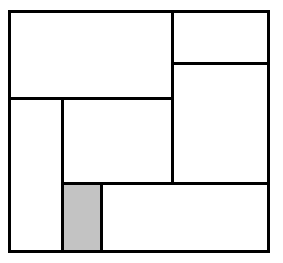But the condition is we are not supposed to rotate the rectangle given. Thus, rectangle D cannot be fitted into it.
Hence, only rectangle A can be fitted into the big main square.

Note: In puzzle games, we cannot rotate the parts of it, if we rotate it and fix, it may or may not be fitted into it, but the picture doesn’t look good as it may be tilted and doesn’t look like the original picture. That is the concept they have used here. Thus we have to adhere to the condition given in the question.